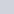Student Forums CMA Part 2 Section E: Investment Decisions E.1. Capital Budgeting Process Question ID: CMA 1278 5.10 (Topic: Capital Budgeting Process)

# Question ID: CMA 1278 5.10 (Topic: Capital Budgeting Process)

• This topic has 3 replies, 2 voices, and was last updated 1 year, 4 months ago byLynn Roden.
• Creator
Topic
• #209462Usama Moosani
Participant

The accountant of Ronier, Inc. has prepared an analysis of a proposed capital project using discounted cash flow techniques. One manager has questioned the accuracy of the results because the discount factors employed in the analysis have assumed the cash flows occurred at the end of the year when the cash flows actually occurred uniformly throughout each year. The net present value calculated by the accountant will
A. Be unusable for actual decision making.
B. Be slightly overstated.
C. Be slightly understated but usable.
D. Not be in error

Can you elaborate the Solution of this Question as I am unable to understand why will the NPV calculated would be Lower than the one calculated considering the Cash Flows were incurred uniformly throughout the year?

Viewing 3 replies - 1 through 3 (of 3 total)
• Author
Replies
• #209474Lynn Roden
HOCK international

Hi Usama,

That is due to the time value of money. The present value of money received earlier is greater than the present value of money that is received later. If money is received at the end of the year (later), its present value is less than if it is received throughout the year (earlier).

So if the money is really received throughout the year but the analysis says it is not received until the end of the year, the analysis will slightly understate the present value of the cash flows.

Lynn

#209488Usama Moosani
Participant

But the Question is askig about the NET PRESENT VALUE and Not the Present Value of Cash Flows. So if the Present Value of Cash Flows is understated, the NPV of Cash Flows would be slightly OVERSTATED.

• This reply was modified 1 year, 4 months ago byUsama Moosani.
#209492Lynn Roden
HOCK international

Hi Usama,

The net present value is the present value of the cash inflows minus the present value of the cash outflows. Usually, the cash outflows all occur at the very beginning of the project, so the initial cash outflow (the initial investment) is not discounted. If the present value of the cash inflows is understated the net present value of the cash flows (the PV of the cash inflows minus the initial investment) will also be understated.

For example, let’s say the initial investment is 100,000. And let’s say the present value of the cash outflows, assuming they are all received at the end of each year, is 250,000. So the net present value is 250,000 – 100,000, which equals 150,000.

If the cash flows are actually received throughout the year, the present value of the cash inflows will be a little higher, so let’s say the actual present value of the cash inflows is 275,000. Now, the net present value is 275,000 – 100,000, or 175,000.

So when we assumed that the cash inflows were all received at the end of each year when they were actually received throughout the year, the net present value is understated because we calculated it as 150,000 when it was actually 175,000.

Lynn

Viewing 3 replies - 1 through 3 (of 3 total)
• You must be logged in to reply to this topic.